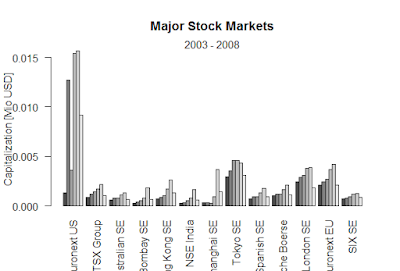Showing posts with label exponents. Show all posts
Showing posts with label exponents. Show all posts

## What is the R language?

R is a programming language and software environment for statistical computing.which is open -source. It is a better tool for statistical analysis.

### We can use R simple calculator.

> 2+2
 4
> 3+2+8
 13
> 0+2
 2

####  -7

> 0-1-3-5-8
 -17
Multiplication
> 2*4*7
 56
> 6*(2*5)*9
 540
Division
> 90/3
 30
> 6789/7
 969.8571
> 4321/9
 480.1111
Trigonometric
> sin(pi/2)
 1
> cos(3*pi/2)
 -1.83691e-16
> tan(pi)
 -1.224647e-16
Logarithm
> log(exp(1))
 1
> log10(1000)
 3
> log2(125)
 6.965784

> log(16, base = 4)

 2
Exponents
> 2^8
 256
> (9+5)^2
 196

### Black-Scholes formula-R

Black-Scholes formula-R > BlackScholes <- function(TypeFlag = c("c", "p"), S, X, Time, r, b, sigma) { TypeFla...